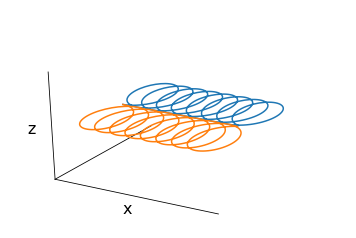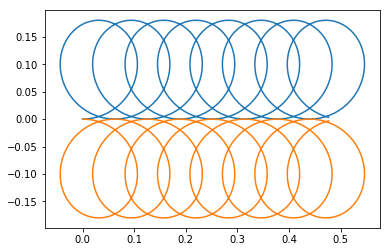## Gyromation of a charged particle in a constant magnetic field¶

### Christian Hill, 11/09/2018¶

In :
import numpy as np
from scipy.integrate import odeint
from matplotlib import animation, rc
rc('animation', html='html5')
import matplotlib.pyplot as plt
from mpl_toolkits.mplot3d import Axes3D

In :
# Magnetic field, electric field
B = np.array((0,0,1))
E = np.array((0,0.01,0))

In :
def lorentz(X, t, q_over_m):
"""
The equations of motion for the Lorentz force on a particle with
q/m given by q_over_m. X=[x,y,z,vx,vy,vz] defines the particle's
position and velocity at time t: F = ma = (q/m)[E + v×B].

"""

v = X[3:]
drdt = v
dvdt = q_over_m * (E + np.cross(v, B))
return np.hstack((drdt, dvdt))

In :
def larmor(q, m, v0):

rho = m.v_perp / (|q|B) where m is the particle's mass,
q is its charge, B is the magnetic field strength and
v_perp is its instantaneous speed perpendicular to the
magnetic field, **B**.

"""

B_sq = B @ B
v0_par = (v0 @ B) * B / B_sq
v0_perp = v0 - v0_par
v0_perp_abs = np.sqrt( v0_perp @ v0_perp)
return m * v0_perp_abs / np.abs(q) / np.sqrt(B_sq)

def calc_trajectory(q, m, r0=None, v0 = np.array((1,0,1))):
"""Calculate the particle's trajectory.

q, m are the particle charge and mass;
r0 and v0 are its initial position and velocity vectors.
If r0 is not specified, it is calculated to be the Larmor
radius of the particle, and particles with different q, m
are placed so as have a common guiding centre (for E=0).

"""
if r0 is None:
rho = larmor(q, m, v0)
vp = np.array((v0,-v0,0))
r0 = -np.sign(q) * vp * rho
# Final time, number of time steps, time grid.
tf = 50
N = 10 * tf
t = np.linspace(0, tf, N)
# Initial positon and velocity components.
X0 = np.hstack((r0, v0))
# Do the numerical integration of the equation of motion.
X = odeint(lorentz, X0, t, args=(q/m,))
return X

In :
def setup_axes(ax):
"""Style the 3D axes the way we want them."""

# Gotta love Matplotlib: this is how to indicate a right-handed
# coordinate system with the x, y, and z axes meeting at a point.
ax.yaxis._axinfo['juggled'] = (1,1,2)
ax.zaxis._axinfo['juggled'] = (1,2,0)
# Remove axes ticks and labels, the grey panels and the gridlines.
for axis in ax.w_xaxis, ax.w_yaxis, ax.w_zaxis:
for e in axis.get_ticklines() + axis.get_ticklabels():
e.set_visible(False)
axis.pane.set_visible(False)
axis.gridlines.set_visible(False)
# Label the x and z axes only.

In :
def plot_trajectories(trajectories):
"""Produce a static plot of the trajectories.

trajectories should be a sequence of (n,3) arrays representing
the n timepoints over which the (x,y,z) coordinates of the
particle are given.

"""

fig = plt.figure()
for X in trajectories:
ax.plot(*X.T[:3])
setup_axes(ax)
# Plot a vertical line through the origin parallel to the
# magnetic field.
zmax = np.max(np.max([X.T for X in trajectories]))
ax.plot([0,0],[0,0],[0,zmax],lw=2,c='gray')
plt.show()

In :
# Test the static plot.

# Electron mass and charge
me, qe = 1, -1
v0 = np.array((0,0,0))
X = calc_trajectory(qe, me, r0=(0,0,0), v0=(0.1,0,0))
X2 = calc_trajectory(-2*qe, me, r0=(0,0,0), v0=(0.1,0,0))
X3 = calc_trajectory(-qe, me, r0=(0,0,0), v0=(0.1,0,0))
plot_trajectories([X, X3])In :
plt.plot(X[:,0], X[:,1])
plt.plot(X3[:,0], X3[:,1])
plt.show()In :
# charges and masses of the electron (e) and ion (i)
qe, me = -1, 1
qi, mi = 1, 3
# Calculate the trajectories for the particles (which don't interact) by
# numerical integration of their equations of motion.
Xe = calc_trajectory(qe, me)
Xi = calc_trajectory(qi, mi)

def init():
"""Initialize the trajectory animation."""

fig = plt.figure()
# The axis of the magentic field
ax.plot([0,0],[0,0],[0,50],lw=2,c='gray')
# Electron motion, ion motion
lne, = ax.plot(*Xe.T[:3])
lni, = ax.plot(*Xi.T[:3])
# The particles instantaneous positions are indicated by circles from
# a scatter plot, scaled according to particle mass. depthshade=0
# ensures that the colour doesn't fade as the the particle's distance
# from the observer increases.
SCALE = 40
particles = ax.scatter(*np.vstack((Xe[:3], Xi[:3])).T,
s=(me*SCALE, mi*SCALE),
# Some tidying up and labelling of the axes.
setup_axes(ax)
return fig, ax, lne, lni, particles

def animate(i):
"""The main animation function, called for each frame."""

def animate_particle(X, ln, i):
"""Plot the trajectory of a particle up to step i."""

ln.set_data(X[:i,0], X[:i,1])
ln.set_3d_properties(X[:i,2])
particles._offsets3d = np.vstack((Xe[i][:3], Xi[i][:3])).T
animate_particle(Xe, lne, i)
animate_particle(Xi, lni, i)

fig, ax, lne, lni, particles = init()
anim = animation.FuncAnimation(fig, animate, frames=500, interval=10, blit=False)

In :
from IPython.display import HTML
HTML(anim.to_html5_video())

Out: